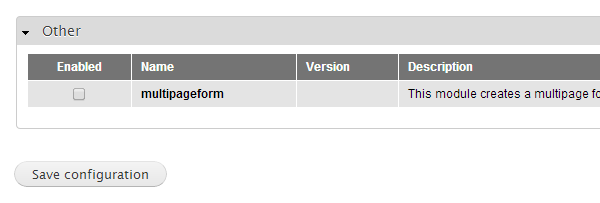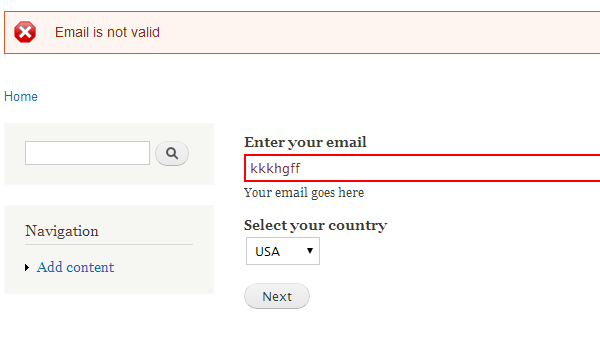# Building a Multi-Page Wizard-like Form in Drupal

Drupal gives you the APIs to build forms by merely specifying the details of the form. It will create the underlying HTML on its own provided you just specify the fields you want in it. We discussed the details of building a form in Drupal in a previous article.

Usally, we would just need a simple form with a few fields, but there may be cases where there might be too many fields on a single page and the form might look confusing and tedious to fill out. This might not make the best UI for your site and it would be good to break your form into logical sections so that it is easier for the user to fill in the information. One way to break the form into different logical blocks is using the field set, but this usually only works if you have a low number of sections. The other way to organize your form is to make it into a wizard or a multi-page form where the user fills the details on one page and then moves ahead to the next page. In this article we will take a look at how to create a multi-page form in Drupal.

## Creating the multipage form module

The first thing we will have to do is create a module in Drupal. To do this, create a folder `sites\all\modules\multipageform` in your Drupal installation and add the following files to it

multipageform.info

``````name = multipageform
description = This module creates a multipage form form using Drupal.
core = 7.x``````

multipageform.module

``````<?php
/**
* @file
* This is the main module file.
*/

/**
* Implements hook_help().
*/
function multipageform_help(\$path, \$arg) {

\$output = '<h3>' . t('About') . '</h3>';
\$output .= '<p>' . t('The multipageform module shows how to create a multiple page form using Drupal.') . '</p>';
return \$output;
}
}``````

Once you have done this you should be able to see the new module in the module list.You can now enable the module.## Defining the number of pages in the form and the form contents.

Once we have our module ready, let’s start defining the Multipage form. To do that, the first thing we will do is create a menu item which will display our form. We will have to add the hook menu in our module as follows

``````/**
*/
\$items['multipageform/form1'] = array(
'access arguments' => array('access content'),
'page callback' => 'drupal_get_form',
'page arguments'=>array('multipageform_form1'));

return \$items;
}

``````

In the above code we have created a menu item at `multipageform/form1` and the page callback is the `drupal_get_form` function with the argument being our `multipageform_form1` function which will return the form for Drupal to render it.

Now we will define the function `multipageform_form1` as follows

``````function multipageform_form1(\$form, &\$form_state) {

if(isset(\$form_state['values'])) {
\$currstep = \$form_state['step'] + 1;
}else {
\$currstep = 0;
}
\$form_state['step'] = \$currstep;
\$allsteps = getForm();
\$currform =  \$allsteps[\$currstep];

return \$currform;
}

function getForm() {

\$form = array();
\$step1 = array();
\$step1['name']=array(
'#type'=>'textfield',
);
\$step1['last_name']=array(
'#type'=>'textfield',
);

\$step1['submit']=array(
'#type'=>'submit',
'#value'=>t('Next')
);

\$form[] = \$step1;

\$step2['email']=array(
'#type'=>'textfield',
);

\$step2['country']=array(
'#type'=>'select',
'#options'=>array('USA','UK','France','Japan')
);

\$step2['submit']=array(
'#type'=>'submit',
'#value'=>t('Next')
);

\$form[] = \$step2;

\$step3['birthdate']=array(
'#type'=>'date',
'#title'=>t('Birthdate'),
);

\$step3['submit']=array(
'#type'=>'submit',
'#value'=>t('Submit')
);

\$form[] = \$step3;

return \$form;
}

``````

In this function the variable `\$form_state` will contain the state of our multipage form. In the state we will store the current step or page we are on, and also the values which have been entered by the user in the previous steps.

The first thing to determine in this function is to know if the user has started a new form or if we need to be on some other step/page of the form. To do this, we will maintain the step we are on in the `\$form_state[‘step’]` variable. If that variable is not set, then we are on step zero. Otherwise, we increment the step by one. Then we store the current step in `\$form_state` for future use.

Next we’ll get a form for the current step. To do this we have to define the form elements for all the steps in a form array. This is done in the function `getForm`. In `getForm` the first step has the name and last name as two form elements; step two has the email and country and step three has a birthday. Here you can easily add or remove elements or even steps according to the form you are trying to build. If you go to the url `<your drupal url>/?q=multipageform/form1` you will see the form as below## Storing the state of the form.

Now we will need to store the state of the form at each stage so that when all the steps are finished, we can store the values in a file or database.

To do that we have to define the function `multipageform_form1_submit` as follows

``````  function multipageform_form1_submit(\$form, &\$form_state) {

\$form_state['storedvalues'][\$form_state['step']] = \$form_state['values'];
if(\$form_state['step'] + 1 != getNumberOfSteps()) {
\$form_state['rebuild'] = TRUE;
}else {

//Reached end of multipage form

}

}

function getNumberOfSteps() {
return count(getForm());
}``````

In the above code the function `getNumberOfSteps` returns the number of steps in the form based on the array of the steps which we have defined. Then in the function `multipageform_form1_submit` we get the values submitted by the user for the current step in `\$form_state['values'];`. We store this value for further use in `form_state` against the current step in the statement

``````\$form_state['storedvalues'][\$form_state['step']] = \$form_state['values'];
``````

Then we check if this step is the last step. If it is not the last step, we set `\$form_state['rebuild']=TRUE` so that the form is rebuilt and our function `multipageform_form1` will generate the form with the next step.

Now if you go to the form URL, enter some values and click next you should move to step two and three as seen below## Multi-page form validation

We might want to validate the data at each step based on the data we are collecting in the form and on some rules we have. To do that we have to write a function `multipageform_form1_validate` as follows

``````  function multipageform_form1_validate(\$form, \$form_state) {

switch (\$form_state['step']) {
case '0':
if(empty(\$form_state['values']['name']))
form_set_error('name','Name cannot be empty');
else if(empty(\$form_state['values']['last_name']))
form_set_error('last_name','Last name cannot be empty');
break;

case '1':
if(filter_var(\$form_state['values']['email'], FILTER_VALIDATE_EMAIL) == false)
form_set_error('email','Email is not valid');
break;

default:
break;
}

}

``````

In the above function the first thing we have to determine is which step we’re on. We do that using `\$form_state['step']` in which we are storing the current step of the form.

Then in the switch block we add cases of all the steps we want some validation in. In the above code we have done validations for step 0 and step 1. In step 0 we check if the name and last name are not empty and in step 1 we check if the email is valid. In case we find an error, we set the error using the Drupal function `form_set_error` which will not move the form to the next step and will show an error to the user:## Multi-page form submission

Once all the steps are complete you will want to extract all the values and then maybe store it in a file or in the database. To do that you will have to modify the `multipageform_form1_submit` function as below

``````function multipageform_form1_submit(\$form, &\$form_state) {

\$form_state['storedvalues'][\$form_state['step']] = \$form_state['values'];
if(\$form_state['step'] + 1 != getNumberOfSteps()) {
\$form_state['rebuild'] = TRUE;
}else {

\$finalformvalues=array();
\$currStep = 0;
foreach (getForm() as \$step) {
foreach (\$step as \$key => \$value) {
if(strcmp(\$key,"submit") != 0) {
\$finalformvalues[\$key] = \$form_state['storedvalues'][\$currStep][\$key];
}
}
\$currStep++;
}
//Store the values from \$finalformvalues in database or file etc

}

}

``````

In the above function, once we detect that we have submitted the last step of the form, we get all the form element keys for all steps and then get the values which the user entered which we had stored in `\$form_state['storedvalues']` before putting them into the array `\$finalformvalues`. Finally `\$finalformvalues` will contain all the values for all form elements for all steps. This can then be further processed and stored.

## Conclusion

Drupal’s Form API lets you create forms without worrying about how to render the HTML for it. Also, we can use the approach shown above to build longer forms which can be broken down into steps so that they are easier and more logical for users to process. Drupal helps us easily carry forward the state in various steps of the form and also provide hooks to validate the values and notify the user in case there are any problems. This all can be done with ease with the functionality that Drupal provides. Have fun creating your next multi-page form in Drupal!